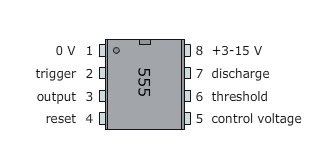## Saturday, January 08, 2011

### IC 555 Monostable Circuit

Monostable circuit produces one pulse of a set length in response to a trigger input Such as a push button. The output of the circuit stays in the low state Until there is a trigger input, Hence the name "monostable" meaning "one stable state".

This type of circuit is ideal for use in a "push to operate" system for a model displayed at EXHIBITIONS. A visitor cans push a button to start a model's mechanism, moving, and the mechanism will from automatically switches off after a set time.

The circuit diagram of the 555 monostable circuit is given as follows.IC 555 Pinout

Note:
• resistor value R and the capacitor value C are unspecified. The values of these components determine the length of time that the monostable output is in the high state, and they may be calculated using the equation below
• T = 1.1RC or R = T/1.1C

In the monostable mode, the timer 555 acts as a "one-shot" pulse generator. The pulse Begins Pls the 555 timer receives a signal at the trigger input That falls below a third of the voltage supply. The width of the output pulse is determined by the time constant of an RC network, the which consists of a capacitor (C) and a resistor (R). The output pulse ends Pls the charge on the C equals 2 / 3 of the supply voltage. The output pulse width cans be lengthened or shortened to the need of the specific application by adjusting the values of R and C

Skema Rangkaian Elektronika